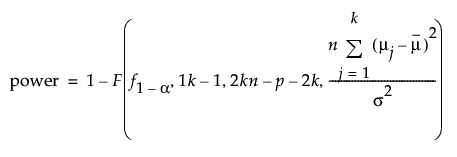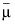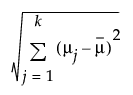Design of Experiments Guide > Prospective Sample Size and Power > k Sample Means Calculator > Statistical Details for the k Sample Means Calculator
Publication date: 05/24/2021

## Statistical Details for the k Sample Means Calculator

The k sample mean calculations are based on the following:where:

α is the significance level

σ is the common standard deviation among the k groups.

n is the sample size per group.

p is the number of extra parameters.is the mean of the k group prospective means

f1-α is the (1 - α)th quantile of the F(k - 1, kn - p - k) distribution.

F(x, df1, df2, nc) is the cumulative distribution function of the non-central F distribution with degrees of freedom df1 and df2 and non-centrality parameter nc evaluated at x.

Numerical solutions are used to solve for n.

#### Difference in Means

The difference in means is calculated as follows: